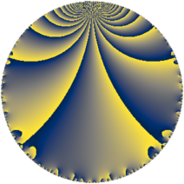# Properties

 Label 42.4.fLevel $42$ Weight $4$ Character orbit 42.f Rep. character $\chi_{42}(5,\cdot)$ Character field $\Q(\zeta_{6})$ Dimension $16$ Newform subspaces $1$ Sturm bound $32$ Trace bound $0$

# Related objects

## Defining parameters

 Level: $$N$$ $$=$$ $$42 = 2 \cdot 3 \cdot 7$$ Weight: $$k$$ $$=$$ $$4$$ Character orbit: $$[\chi]$$ $$=$$ 42.f (of order $$6$$ and degree $$2$$) Character conductor: $$\operatorname{cond}(\chi)$$ $$=$$ $$21$$ Character field: $$\Q(\zeta_{6})$$ Newform subspaces: $$1$$ Sturm bound: $$32$$ Trace bound: $$0$$

## Dimensions

The following table gives the dimensions of various subspaces of $$M_{4}(42, [\chi])$$.

Total New Old
Modular forms 56 16 40
Cusp forms 40 16 24
Eisenstein series 16 0 16

## Trace form

 $$16 q + 32 q^{4} + 80 q^{7} + 18 q^{9} + O(q^{10})$$ $$16 q + 32 q^{4} + 80 q^{7} + 18 q^{9} - 36 q^{10} - 128 q^{16} - 48 q^{18} - 342 q^{19} - 450 q^{21} + 24 q^{22} - 48 q^{24} - 194 q^{25} + 88 q^{28} + 360 q^{30} + 804 q^{31} + 1332 q^{33} + 144 q^{36} - 962 q^{37} + 594 q^{39} - 144 q^{40} - 180 q^{42} + 1732 q^{43} - 2394 q^{45} + 168 q^{46} + 820 q^{49} + 1638 q^{51} + 744 q^{52} + 180 q^{54} - 2664 q^{57} - 780 q^{58} - 4620 q^{61} - 2016 q^{63} - 1024 q^{64} - 2016 q^{66} - 706 q^{67} - 60 q^{70} + 192 q^{72} + 3294 q^{73} + 6174 q^{75} + 2832 q^{78} - 2656 q^{79} + 126 q^{81} + 432 q^{82} - 432 q^{84} + 5232 q^{85} + 1026 q^{87} + 48 q^{88} + 4098 q^{91} + 2016 q^{93} + 3888 q^{94} - 192 q^{96} - 4284 q^{99} + O(q^{100})$$

## Decomposition of $$S_{4}^{\mathrm{new}}(42, [\chi])$$ into newform subspaces

Label Dim $A$ Field CM Traces $q$-expansion
$a_{2}$ $a_{3}$ $a_{5}$ $a_{7}$
42.4.f.a $16$ $2.478$ $$\mathbb{Q}[x]/(x^{16} - \cdots)$$ None $$0$$ $$0$$ $$0$$ $$80$$ $$q-\beta _{2}q^{2}+\beta _{1}q^{3}+(4+4\beta _{5})q^{4}+(-\beta _{2}+\cdots)q^{5}+\cdots$$

## Decomposition of $$S_{4}^{\mathrm{old}}(42, [\chi])$$ into lower level spaces

$$S_{4}^{\mathrm{old}}(42, [\chi]) \cong$$ $$S_{4}^{\mathrm{new}}(21, [\chi])$$$$^{\oplus 2}$$